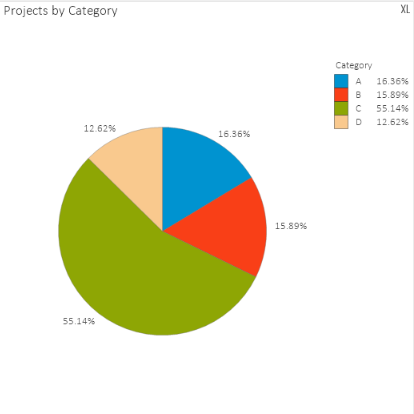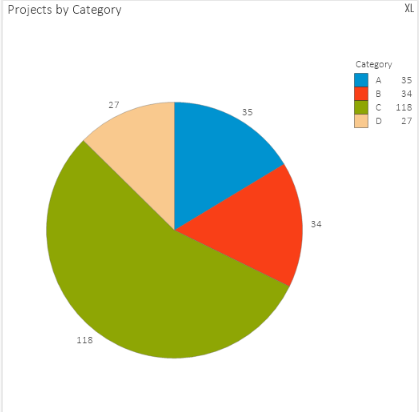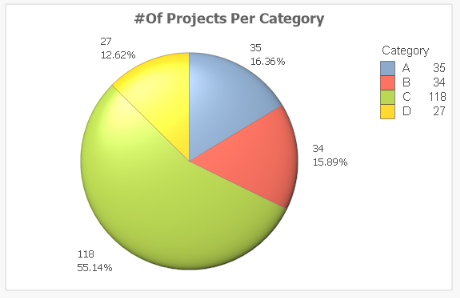# New to QlikView

Discussion board where members can get started with QlikView.

Highlighted
Contributor III

## How to show Count and Percentage on the Pie chart visual ?

Hello,

I have the below Pie Chart which shows the No of Projects per Category;When I uncheck the relative under my expression I am getting the count of the projects as shown below;How Can I get both percentages and count of the projects in my Pie chart visual? any help is greatly appreciated.

thanks

Bhavesh

Tags (2)
1 Solution

Accepted Solutions
Contributor III

## Re: How to show Count and Percentage on the Pie chart visual ?

Couple of answers that work here, I find the easiest option to be to simply add a second expression with the exact same expression (for example Count(Project)), and tick relative for one of the expressions and leave it off for the other. Check Value on data points for both and you should have what you want. Example:You won't be able to see the percentage in the legend though, you'll have to use Nassim's solution for that.

4 RepliesPartner

## Re: How to show Count and Percentage on the Pie chart visual ?

You need to use dual function.

something like

dual( your_sum_expression & '  - ' & your_percent_expression,your_sum_expression)

something like

dual(Num(Sum(Sales),'0,000.00') & '-' & num((Sum(Sales)/Sum(TOTAL Sales))*100,'0.00') & '%',

Num(Sum(Sales),'0,000.00'))

New Contributor III

## Re: How to show Count and Percentage on the Pie chart visual ?

Hi,

just add a calculated dimension  as

=Category&' : '& aggr(num(sum(#Projects)/sum(total{<Category>} #Projects),'#,##0.00%'),Category)

and the expression is :

sum(#Projects)

and don't select relative check box .

thanks

nassim

Contributor III

## Re: How to show Count and Percentage on the Pie chart visual ?

Couple of answers that work here, I find the easiest option to be to simply add a second expression with the exact same expression (for example Count(Project)), and tick relative for one of the expressions and leave it off for the other. Check Value on data points for both and you should have what you want. Example:You won't be able to see the percentage in the legend though, you'll have to use Nassim's solution for that.

Contributor III

## Re: How to show Count and Percentage on the Pie chart visual ?

That was the easiest thing to do. thank you